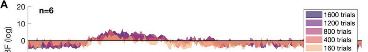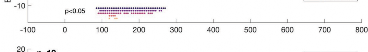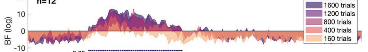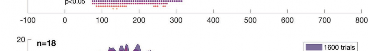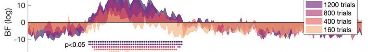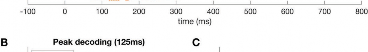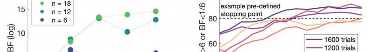# An Empirically Driven Guide on Using Bayes Factors for M/EEG Decoding

#### Lina Teichmann¹,*, Denise Moerel², Chris Baker¹, Tijl Grootswagers³

¹ Laboratory of Brain and Cognition, National Institute of Mental Health, Bethesda, MD, USA ² Department of Cognitive Science, Macquarie University, Sydney, Australia

³ The MARCS Institute for Brain, Behaviour & Development, Western Sydney University, Sydney, Australia

## ABSTRACT

Bayes factors can be used to provide quantifiable evidence for contrasting hypotheses and have thus become increasingly popular in cognitive science. However, Bayes factors are rarely used to statistically assess the results of neuroimaging experiments. Here, we provide an empirically driven guide on implementing Bayes factors for time-series neural decoding results. Using real and simulated magnetoencephalography (MEG) data, we examine how parameters such as the shape of the prior and data size affect Bayes factors. Additionally, we discuss the benefits Bayes factors bring to analysing multivariate pattern analysis data and show how using Bayes factors can be used instead or in addition to traditional frequentist approaches.

Acknowledgements: This research was supported (in part) by the Intramural Research Program of the NIMH (ZIAMH002909). We thank Lincoln Colling for contributing to the repository.

Date Accepted: January 16, 2022

## INTRODUCTION

The goal of multivariate decoding in cognitive neuroscience is to infer whether information is represented in the brain (Hebart & Baker, 2018). To draw meaningful conclusions in this information-based framework, we need to statistically assess whether the conditions of interest evoke different data patterns. In the context of time-resolved neuroimaging data, activation patterns are extracted across magnetoencephalography (MEG) or electroencephalography (EEG) sensors, and classification accuracies are used to estimate information at every timepoint (see Figure 1 for an example). Currently, null hypothesis statistical testing (NHST) and p-values are the de facto method of choice for statistically assessing classification accuracies, but recent studies have started using Bayes factors (Grootswagers et al., 2021; e.g., Grootswagers et al., 2019b; Grootswagers, Robinson, Shatek, et al., 2019; Kaiser et al., 2018; Karimi-Rouzbahani et al., 2021; Mai et al., 2019; Proklova et al., 2019; Robinson et al., 2019, 2021). Bayes factors describe the probability of one hypothesis over the other given the observed data. In the multivariate pattern analysis (MVPA) context, we use

Bayes factors to test the probability of above-chance classification versus at-chance classification given the decoding results across participants at each timepoint. The direct comparison of the predictions of two hypotheses is one of the strengths of the Bayesian framework of hypothesis testing (Jeffreys, 1935, 1939). The goal of this paper is to present and discuss Bayes factors from a practical standpoint in the context of time-series decoding, while referring the reader to published work focusing on the theoretical and technical background of Bayes factors.

The Bayesian approach brings several advantages over the traditional NHST framework (Dienes, 2011, 2014, 2016; Keysers et al., 2020; Morey et al., 2016; Wagenmakers et al., 2018). In addition to allowing us to contrast evidence for above-chance versus at-chance decoding directly, Bayes factors are a measure of strength of evidence for one hypothesis versus another. That means, we can directly assess how much evidence we have for different analyses. For example, if we were interested in testing whether viewing different colours evokes different neural responses, we could examine differences in the neural signal evoked by seeing red, green, and yellow objects. Using Bayes factors, we could then directly compare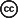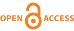Teichmann et al. This is an open access article distributed under the terms of the Creative Commons Attribution-NonCommercial-NoDerivs 4.0 IGO License, which permits the copy and redistribution of the material in any medium or format provided the original work and author are properly credited. In any reproduction of this article there should not be any suggestion that APERTURE NEURO or this article endorse any specific organization or products. The use of the APERTURE NEURO logo is not permitted. This notice should be preserved along with the article’s original URL. Open access logo and text by PLoS, under the Creative Commons Attribution-Share Alike 4.0 Unported license.

O R I G I N A L R E S E A R C H A R T I C L E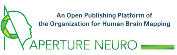O R I G I N A L R E S E A R C H A R T I C L E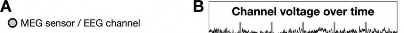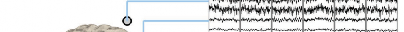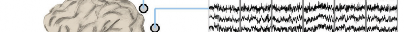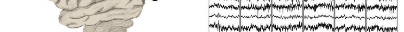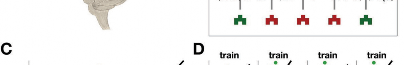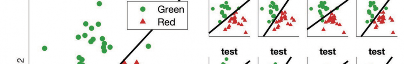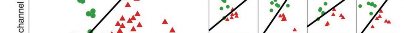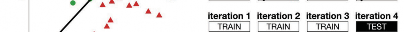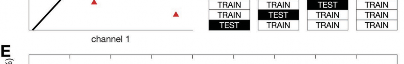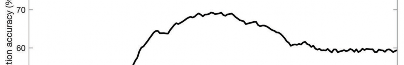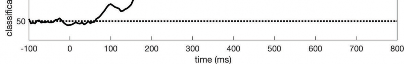Fig. 1. Overview of MVPA for time-series neural data. (A) Example MEG sensors/EEG channels. (B) Simulated time-series neuroimaging data for a few sensors/ channels. Vertical lines show stimulus onsets with example stimuli plotted below. Data are first epoched from −100 ms to 800 ms relative to stimulus onset, resulting in multiple time-series chunks associated with seeing a red or a green shape. (C) Using the epoched data, we can extract the sensor/channel activation pattern across the different sensors/channels (only 2 displayed for simplicity) for every trial at every timepoint. Then a classifier (black line) is trained to differentiate between the activation patterns evoked by red and green trials. The shape of the stimuli is not relevant in this context. (D) Example of a 4-fold cross validation where the classifier is trained on three quarters of the data and tested on the left-out quarter. This process is repeated at everytimepoint. (E) We can calculate how often the classifier accurately predicts the colour of the stimulus at each timepoint by averaging across all testing folds. Theoretical chance level is 50% as there are two conditions in the simulated data (red and green). During the period before stimulus onset, we expect decoding to be at chance, and thus the baseline period can serve as a sanity check.

whether red versus green can be decoded as well as red versus yellow. Larger Bayes factors reflect more evidence that makes the interpretation of statistical results across analyses more intuitive. Another advantage is that Bayes factors can be calculated iteratively while more data are being collected and that testing can be stopped when there is a sufficient amount of evidence (Keysers et al., 2020; Wagenmakers et al., 2018). Such stopping rules could be accompanied by a pre-specified acquisition plan and potentially an (informal) pre-registration via portals such as the Open Science Framework (Foster & Deardorff, 2017).

Using the data to determine when enough evidence has been collected is particularly relevant for

neuroimaging experiments, as it might significantly reduce research costs and reduce the risk of having underpowered studies. Thus, using a Bayesian approach to statistically assess time-series classification results can be beneficial both from a theoretical as well as an economic standpoint and might ease the ability to interpret and communicate scientific findings.

While Bayes factors provide an alternative to the more traditional NHST framework, incorporating Bayes factors into existing time-series decoding pipelines may seem daunting. Introductory papers often focus on mathematical aspects and on relatively straightforward behavioural experiments (e.g., Ly et al., 2016; Morey et al., 2016; Rouder et al., 2009). We present an example

based on a previously published time-series decoding study (Teichmann et al., 2019) and will present results from simulations to show the influence of certain parameters on Bayes factors. We make use of the established Bayes Factor R package (Morey et al., 2015) to calculate the Bayes factors but provide sample codes along with this paper showing how to access the Bayes Factor R package via Matlab and Python (https://github. com/LinaTeichmann1/BFF_repo). We also show how the Bayes factors in our example compare to p-values. Based on empirical evidence, we will give recommendations for Bayesian analysis applied to M/EEG classification results. The aim of this paper is to provide a broad introduction to Bayes factors from a viewpoint of time-series neuroimaging decoding. We aim to do so without going into the technical or mathematical detail and instead provide pointers to relevant literature on the specifics.

## METHODS AND RESULTS

### Example dataset and inferences based on Bayes factors

The aim of the current paper is to show how to use Bayes factors when assessing time-series neuroimaging classification results and test what effect different analysis parameters have on the results. We have used a practical example of previously published MEG data (Teichmann et al., 2019), which we re-analysed using Bayes factors. In the original experiment, participants viewed coloured shapes and grayscale objects in separate blocks while

O R I G I N A L R E S E A R C H A R T I C L E

the neural signal was recorded using MEG. Here, we only considered the coloured shape trials (“real colour blocks”, 1600 trials in total). Identical shapes were coloured in red or green and were shown for 100 ms followed by an inter-stimulus interval of 800–1100 ms. The data were epoched from −100 ms to 800 ms (200 Hz resolution) relative to stimulus onset and a linear classifier was used to differentiate between the neural responses evoked by red and green shapes. A 5-fold cross-valida-tion was used with the classifier being trained on 80% of the data and tested on the remaining 20%. This classification analysis resulted in decoding accuracies over time for each participant. In the original study, permutation tests and cluster-corrected p-values were used to assess decoding accuracies as implemented in CoSMoMVPA (Oosterhof et al., 2016). Here, we calculated Bayes factors instead and examined how parameter changes affected the results.

When running statistical tests on classification results, we are interested in whether decoding accuracy is above chance at each timepoint. To test this using a frequentist approach, we can use permutation tests to establish whether there is enough evidence to reject H, which states that decoding is equal to chance. If there is enough evidence, we can reject Hand conclude that decoding is different from chance. Given that below-chance decoding accuracies are not meaningful, we usually are interested only in above-chance decoding (directional hypothesis).

In contrast to the frequentist approach, Bayes factors quantify how much the plausibility of two hypotheses changes, given the data (see e.g., Ly et al., 2016). Here, we ran a Bayesian t-test of Bayes Factor R package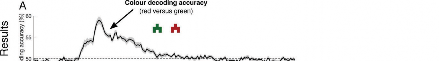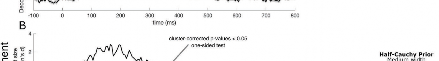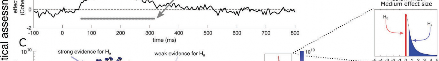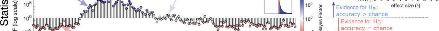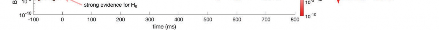Fig. 2. Decoding results of our practical example dataset with statistical assessments. (A) Colour decoding over time (black line). The dashed line shows theoretical chance decoding (50%). The grey-shaded area represents the standard error across participants. (B) Effect size over time with the cluster-corrected p-values at each timepoint printed below in grey. (C) Bayes factors over time for this dataset on a logarithmic scale. Blue, upwards pointing stems indicate evidence for above-chance decoding and red, downwards pointing stems show evidence for at-chance decoding at every timepoint. We used a hybrid one-sided model comparing evidence for above-chance decoding versus a point-nil at 𝛿 = 0 (no effect). For the alternative hypothesis, we used a half-Cauchy prior with medium width (r = 0.707) covering an interval from 𝛿 = 0.5 to 𝛿 = ∞. The half-Cauchy prior assumes that small effect sizes are more likely than large ones, but the addition of the interval deems very small effects 𝛿

< 0.5 as irrelevant. During the baseline period (i.e., before stimulus onset), the Bayes factors strongly support the null hypothesis, confirming the sanity check expectation.

O R I G I N A L R E S E A R C H A R T I C L E

(Morey et al., 2015) at each timepoint, testing whether the data are more consistent with H(decoding is larger than chance) over H(decoding is equal to chance). The resulting Bayes factors centre around 1 with numbers smaller than 1 representing evidence for Hand numbers larger than 1 representing evidence for H. In contrast to p-values, Bayes factors are directly interpretable and comparable (cf. Keysers et al., 2020; Morey et al., 2016; Wagenmakers et al., 2016). That is, a Bayes factor of 10 means that the data are 10 times more likely to be observed under Has opposed to H. Similarly, a Bayes factor of 1/10 means that the data are 10 times more likely to be observed under Has opposed to H. Thus, in the context of time-series decoding, Bayes factors allow us to directly assess whether and how much evidence there is at a given timepoint for the alternative over the null hypothesis and vice versa (Figure 2C).

### Adjusting the prior range to account for observed chance decoding

Bayes factors represent the plausibility that the data emerged from one hypothesis compared to another. In the example dataset, the two hypotheses are that decoding is at chance (i.e., H, no colour information present) or that decoding is above chance (i.e., H, colour information present). To deal with the fact that observed chance decoding can be different than the theoretical chance level, we can adjust the prior range of the alternative hypothesis to allow for small effects under the null hypothesis (Morey & Rouder, 2011). The prior range (called “null interval” in the R package) is defined in standardized effect sizes and consists of a lower and upper bound. To incorporate the differences between observed and theoretical chance level, we can define a range of relevant effect sizes for the alternative hypothesis, for example, from 𝛿 = 0.5 to 𝛿 = ∞. To determine which values are reasonable as the lower bound of this interval, we changed

the prior range systematically and examined the effect on the resulting Bayes factors (Figure 3). We found that smaller lower bounds at 𝛿 = 0 and 𝛿 = 0.2 resulted in weaker evidence supporting the null hypothesis than ranges starting at 𝛿 = 0.5 and 𝛿 = 0.8.

The range did not have a large effect on timepoints with strong evidence for H. The effect of changing the prior range is larger for the null hypothesis than the alternative as chance decoding is not exactly 50% but distributed around chance. Changing the lower bound of the prior range means that the effects that are just larger than 𝛿 = 0 can support the null hypothesis. Thus, the results here demonstrate that we can compensate for the differences between theoretical and observed chance by adjusting the prior range and effectively considering small effect sizes as evidence for the null hypothesis rather than the alternative.

To further examine what a reasonable lower bound of the prior range is, we looked at effect sizes observed during the baseline window (before stimulus onset) in a selection of our previous studies (Grootswagers et al., 2021; Grootswagers et al., 2019a; Moerel, Grootswagers et al., 2021; Moerel, Rich, et al., 2021; Teichmann et al., 2018, 2020). Using the baseline window allows us to quantify the difference between theoretical and observed chance, as we do not expect any meaningful effects before stimulus onset (e.g., stimulus colour is not decodable before the stimulus is presented). Thus, the baseline period can effectively tell us which effect sizes can be expected by chance. Using this method, we estimated maximum effect sizes for different analyses in each paper (see different bars in Figure 4). Across our selection of prior studies, we found an average maximum effect size of 𝛿 = 0.39 before stimulus onset and an average maximum effect size of 𝛿 = 1.91 after stimulus onset (Figure 4). This survey shows that effect sizes as large as 𝛿 = 0.5 can be observed when no meaningful information is in the signal. Thus, this supports the conclusions from the example dataset showing that prior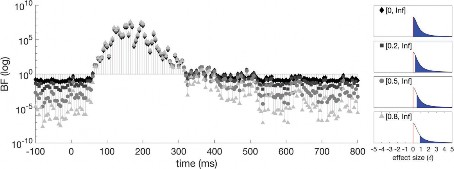Fig. 3. The effect of changing the prior range (null interval) on Bayes factors in our example data. Intervals starting at larger effect sizes led to more timepoints showing conclusive evidence for H. This is due to the fact that theoretical and observed chance levels are not the same. The panels on the right show the prior distributions with the different null intervals.

O R I G I N A L R E S E A R C H A R T I C L E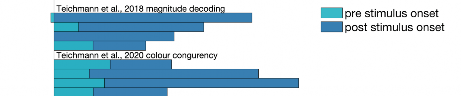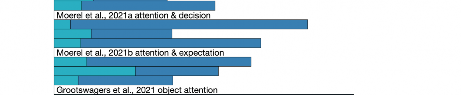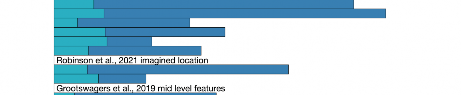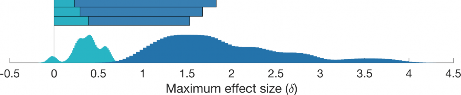Fig. 4. Estimated maximum effect sizes during baseline and after stimulus onset for prior decoding studies that used visual stimuli. Using already published data, we calculated the maximum effect sizes during the baseline (light blue) and post-stimulus (dark blue) to estimate typical peak effect sizes in visual decoding studies. Each bar represents a unique analysis within the paper. The estimations show that a reasonable range for Hwould start at 𝛿 = 0.5 or above, as during baseline decoding accuracies corresponding to standardized effect sizes as high as 𝛿 = 0.5 were observed.

ranges with a lower bound of 𝛿 = 0.5 may be a sensible choice when using Bayes factors to examine time-series M/EEG decoding results.

### Changing the prior width to capture different effect sizes

Another feature that can be changed in the Bayesian t-test is the width of the half-Cauchy distribution (referred to as r-value in the Bayes Factor package). Small r-values create a narrower, sharply peaking distribution, whereas larger values make the distribution wider with a prolonged peak. Standard prior widths incorporated in the Bayes Factor R package are medium (r = 0.707), wide (r = 1), and ultrawide (r = 1.414). Keeping the prior range consistent ([0.5, Inf]) while using the three prior widths implemented into the R Bayes Factor package (medium = 0.707; wide = 1; ultrawide = 1.414). We found that changing the width of the Cauchy prior did not have a pronounced effect on the Bayes factors (Figure 5). In our specific example, this is probably the case because the effect sizes quickly rose to

𝛿 > 2 (Figure 2b), which means that the subtle differences between the different prior widths do not have a substantial effect on the likelihood of the data arising from Hover H. Thus, using the default prior width (r = 0.707) for the decoding context seems like a reasonable choice.

### The effect of data size on statistical inferences

In a lot of cases, there are financial and time limits on how many participants can be tested and for how long. To obtain an estimate of how much data are needed to draw conclusions and avoid ending up with underpowered studies, we used the example dataset and reduced the data size for analysis. As classification analyses are usually run at the subject level but statistical assessment is run at the group level, we tested how changing data size both by trial numbers and participant numbers influences Bayes factors in the time-series decoding context (Figure 6). In the original example dataset, the classifier was trained on 1408 trials and tested on 352 trials (5-fold cross-validation). There were five different shapes in the red and the green condition (160 repetitions for each coloured shape), and the cross-valida-tion schema was based on leaving all trials of one shape out for testing. Statistical inferences were drawn on the group level that contained data from 18 participants. To examine the effect of data size (and effectively noise level) on the Bayes factor calculations, we re-ran the analysis reducing the data size first by retaining the first 1200 (75%), 800 (50%), 400 (25%), or 160 (10%) trials participants completed. We cross-validated in the same way as in the original paper, with the only difference being how many trials of each shape were included. In addition, we subsampled from

O R I G I N A L R E S E A R C H A R T I C L E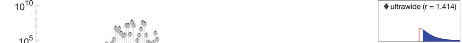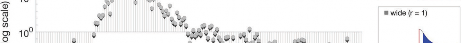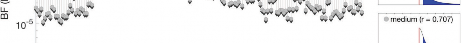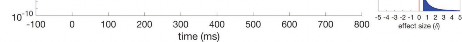Fig. 5. Bayes factors over time for the example dataset when the prior width is changed. The width of the prior had no pronounced effect on the Bayes factors we calculated. The panels on the right show the prior distributions with the different widths.